# 5. (2pts) To calculate the pH of buffer solution we need to use Henderson-Hasselbalch equation The...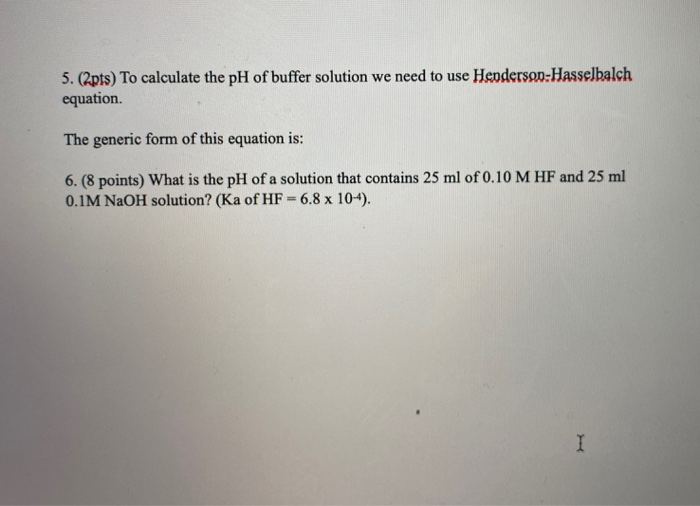5. (2pts) To calculate the pH of buffer solution we need to use Henderson-Hasselbalch equation The generic form of this equation is: 6. (8 points) What is the pH of a solution that contains 25 ml of 0.10 M HF and 25 ml 0.1M NaOH solution? (Ka of HF -6.8 x 104).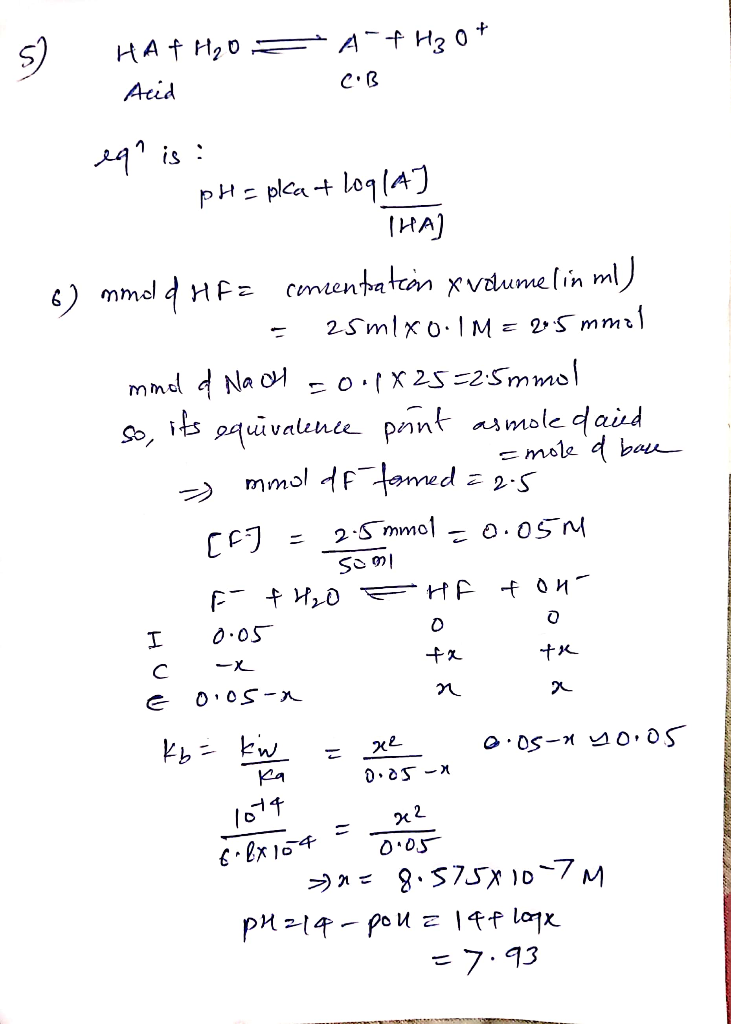#### Earn Coin

Coins can be redeemed for fabulous gifts.

Similar Homework Help Questions
• ### Use the Henderson-Hasselbalch equation to calculate the pH of each solution: (a) a solution that is...

Use the Henderson-Hasselbalch equation to calculate the pH of each solution: (a) a solution that is 0.20 M in HCHO2 and 0.10 M in NaCHO2. (Ka=1.8×10−4) (b) a solution that is 0.14 M in NH3 and 0.19 M in NH4Cl. (Kb=1.76×10−5)

• ### 1. (3) Using the Henderson-Hasselbalch Equation, calculate the pH of a buffer solution that is 0.065...1. (3) Using the Henderson-Hasselbalch Equation, calculate the pH of a buffer solution that is 0.065 M in benzoic acid (HC2H5O2) and 0.125 M is sodium benzoate (NaC7H5O2). For benzoic acid, Ka = 6.5 x 105.

• ### Use the Henderson-Hasselbalch equation to calculate the pH of each of the following solutions. A. a...

Use the Henderson-Hasselbalch equation to calculate the pH of each of the following solutions. A. a solution that contains 0.800% C5H5N by mass and 0.950% C5H5NHCl by mass (where pKa=5.23 for C5H5NHCl B. a solution that has 17.0 g g of HF and 27.0 g g of NaF in 125 mL m L of solution (where pKa=3.17 for HF acid)

• ### HELP!!! The Henderson-Hasselbalch (Buffer) Equation • The pH of the buffer solution is dependent more on...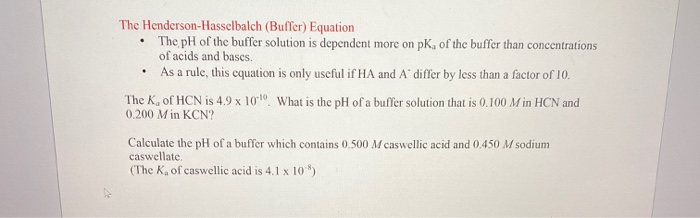HELP!!! The Henderson-Hasselbalch (Buffer) Equation • The pH of the buffer solution is dependent more on pk, of the buffer than concentrations of acids and bases • As a rule, this equation is only useful if HA and A differ by less than a factor of 10 The K, of HCN is 4.9 x 10 What is the pH of a buffer solution that is 0.100 Min HCN and (0.200 M in KCN? Calculate the pH of a buffer which...

• ### Use the Henderson-Hasselbalch equation to calculate the mass of solid sodium acetate required to mix with...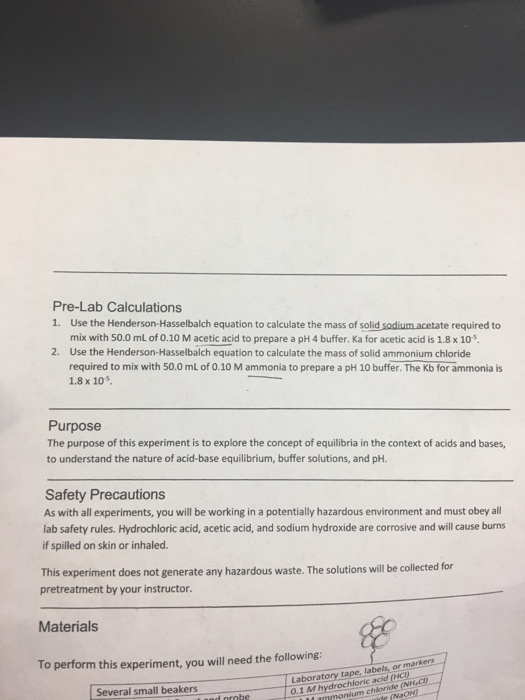Use the Henderson-Hasselbalch equation to calculate the mass of solid sodium acetate required to mix with 50.0 mL of 0.10 M acetic acid to prepare a pH 4 buffer. Ka for acetic acid is 1.8 times 10^-5. Use the Henderson-Hasselbalch equation to calculate the mass of solid ammonium chloride required to mix with 50.0 mL of 0.10 M ammonia to prepare a pH 10 buffer. The Kb for ammonia is 1.8 times 10^-5. The purpose of this experiment is to...

• ### Using Henderson-Hasselbalch equation, Calculate the pH of a buffer solution that is 0.060 M formic acid...

Using Henderson-Hasselbalch equation, Calculate the pH of a buffer solution that is 0.060 M formic acid (HCHO2) and 0.150 M potassium formate (KCHO2). Remember that Ka = 1.8 X 10-4 for formic acid. Group of answer choices A 1.45 B 2.36 C.9.12 D.4.13 E. 0.0125 F. 7.00

• ### 5. You are asked to prepare an acetate buffer solution at pH 4.6. Use the Henderson-Hasselbalch...5. You are asked to prepare an acetate buffer solution at pH 4.6. Use the Henderson-Hasselbalch equation to calculate the ratio of sodium acetate and acetic acid required to prepare a buffer at pH 4.6. 6. If you had 500mL of 0.200M sodium acetate buffer pH4.76, how many mL of 1.00M HCI would you need to add to adjust the pH to 4.60?

• ### The Henderson-Hasselbalch equation relates the pH of a buffer solution to the pKa of its conjugat...

The Henderson-Hasselbalch equation relates the pH of a buffer solution to the pKa of its conjugate acid and the ratio of the concentrations of the conjugate base and acid. The equation is important in laboratory work that makes use of buffered solutions, in industrial processes where pH needs to be controlled, and in medicine, where understanding the Henderson-Hasselbalch equation is critical for the control of blood pH. Part A As a technician in a large pharmaceutical research firm, you need...

• ### pH of 9.2 using ammonium nitrate: pKa= 9.24 2 Introduiction The Henderson-Hasselbalch equation, or A-1 HAl relates the pH of a buffer with the pKo of the acid and the concentration of the conju...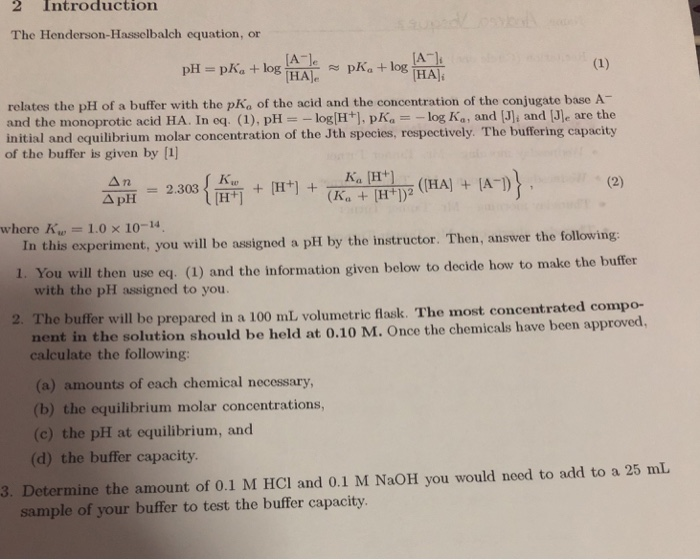pH of 9.2 using ammonium nitrate: pKa= 9.24 2 Introduiction The Henderson-Hasselbalch equation, or A-1 HAl relates the pH of a buffer with the pKo of the acid and the concentration of the conjugate base A- and the monoprotic acid HA. In cq. (1), pH-_ log[H+], pK, =-log Ka, and [J]i and [JIe are the initial and equilibrium molar concentration of the Jth species, respectively. The buffering capacity of the buffer is given by  Ka H+ where K,e-1.0 ×...

• ### Use the Henderson-Hasselbalch Equation to calculate the pH of a buffer solution that 10 0.050 Min...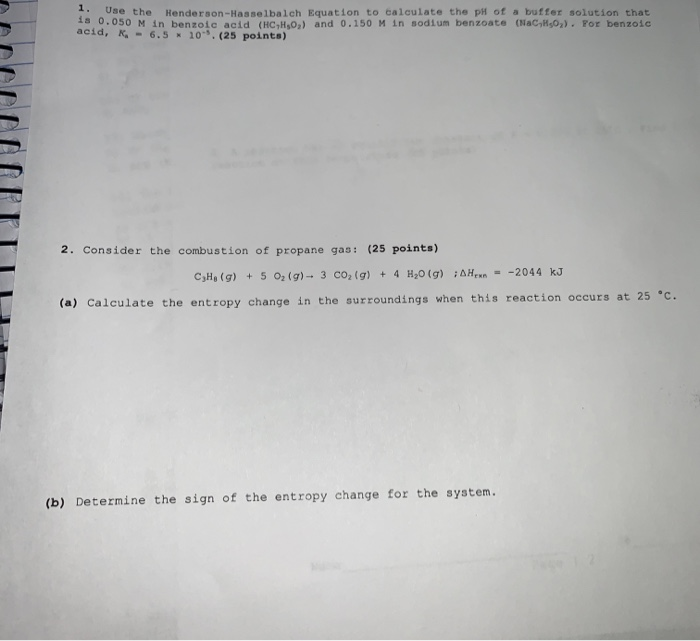Use the Henderson-Hasselbalch Equation to calculate the pH of a buffer solution that 10 0.050 Min benzoic acid (HC,1,0,) and 0.150 Min sodium benzoate ac or benzoic acid, K, - 6.5 * 10. (25 points) 2. Consider the combustion of propane gas: (25 points) CH, (g) + 5 0,(9) -- 3 CO2(g) + 4 H20 (g) ; AHU - -2044 kJ (a) Calculate the entropy change in the surroundings when this reaction occurs at 25 °C. (b) Determine the sign...